## Batter up

The movie Moneyball focuses on the “quest for the secret of success in baseball”. It follows a low-budget team, the Oakland Athletics, who believed that underused statistics, such as a player’s ability to get on base, betterpredict the ability to score runs than typical statistics like home runs, RBIs (runs batted in), and batting average. Obtaining players who excelled in these underused statistics turned out to be much more affordable for the team.

In this lab we’ll be looking at data from all 30 Major League Baseball teams and examining the linear relationship between runs scored in a season and a number of other player statistics. Our aim will be to summarize these relationships both graphically and numerically in order to find which variable, if any, helps us best predict a team’s runs scored in a season.

## The data

Let’s load up the data for the 2011 season.

load("more/mlb11.RData")

In addition to runs scored, there are seven traditionally used variables in the data set: at-bats, hits, home runs, batting average, strikeouts, stolen bases, and wins. There are also three newer variables: on-base percentage, slugging percentage, and on-base plus slugging. For the first portion of the analysis we’ll consider the seven traditional variables. At the end of the lab, you’ll work with the newer variables on your own.

1. What type of plot would you use to display the relationship between runs and one of the other numerical variables? Plot this relationship using the variable at_bats as the predictor. Does the relationship look linear? If you knew a team’s at_bats, would you be comfortable using a linear model to predict the number of runs?

I would use a scatter plot to display the relationship between the variables.

plot(mlb11$at_bats, mlb11$hits,  # plot the variables
xlab="At Bats",              # x−axis label
ylab="Hits")                 # y−axis label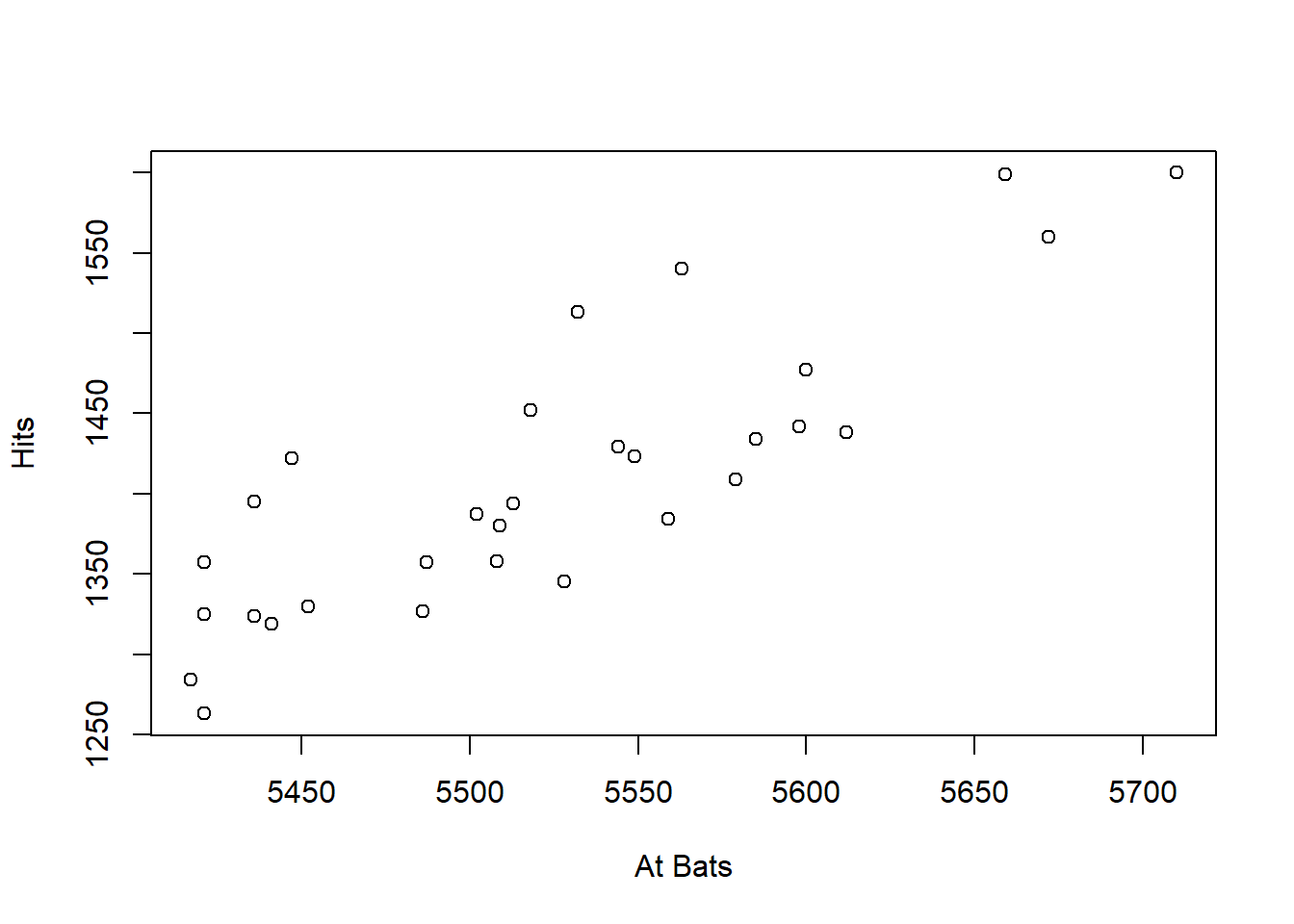Yes, the relationship looks linear.

Yes, If I knew a team’s at_bats, I would be comfortable using a linear model to predict the number of runs.

If the relationship looks linear, we can quantify the strength of the relationship with the correlation coefficient.

cor(mlb11$runs, mlb11$at_bats)
##  0.610627

## Sum of squared residuals

Think back to the way that we described the distribution of a single variable. Recall that we discussed characteristics such as center, spread, and shape. It’s also useful to be able to describe the relationship of two numerical variables, such as runs and at_bats above.

1. Looking at your plot from the previous exercise, describe the relationship between these two variables. Make sure to discuss the form, direction, and strength of the relationship as well as any unusual observations.
plot(mlb11$at_bats, mlb11$runs,  # plot the variables
xlab="At Bats",              # x−axis label
ylab="Runs")                 # y−axis label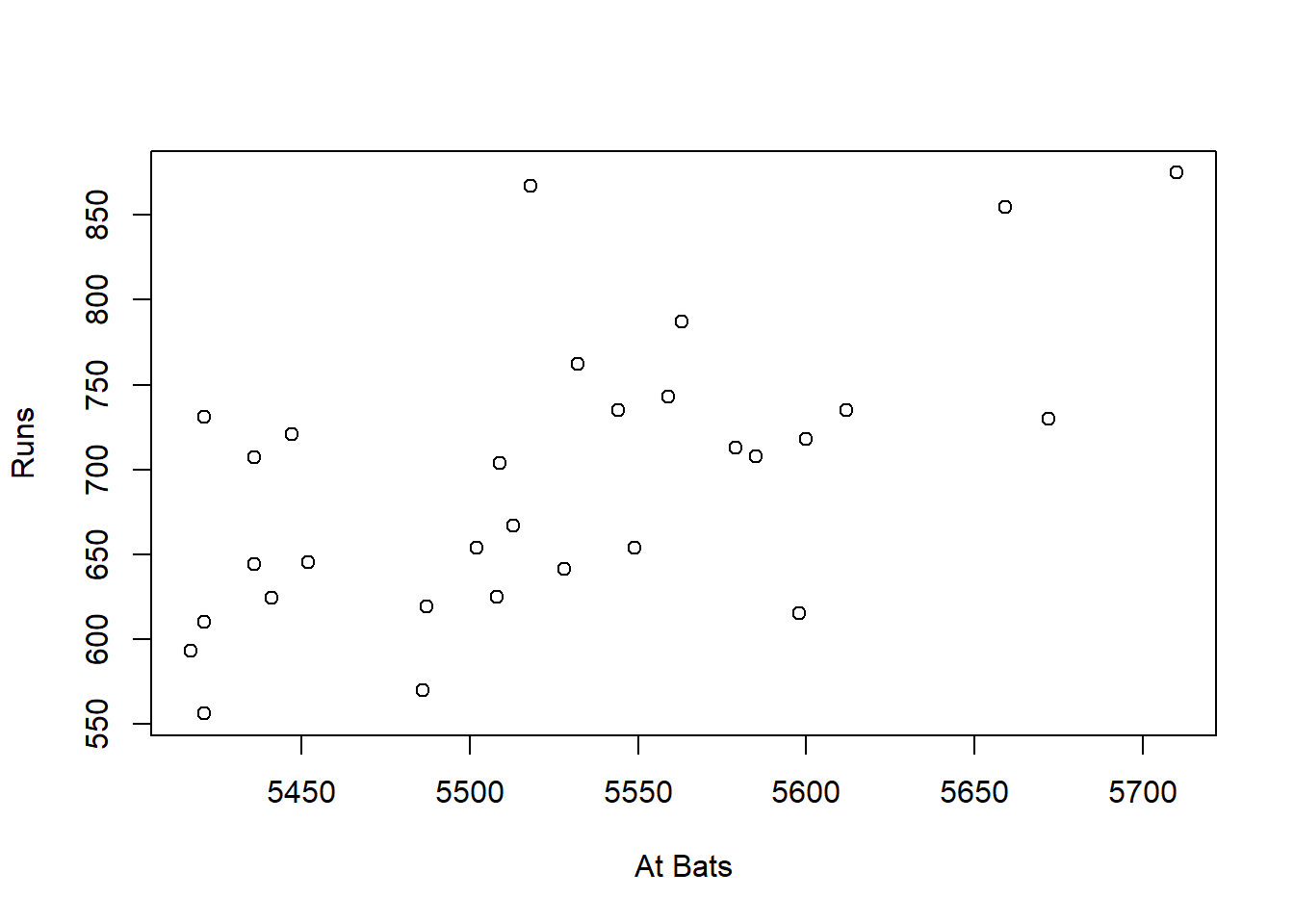The relationship seems to be a little sparsed or dispersed, the distribution of the points seems to grow in a positive direction with some leverage and the relationship seems to have a positive correlation.

Just as we used the mean and standard deviation to summarize a single variable, we can summarize the relationship between these two variables by finding the line that best follows their association. Use the following interactive function to select the line that you think does the best job of going through the cloud of points.

plot_ss(x = mlb11$at_bats, y = mlb11$runs)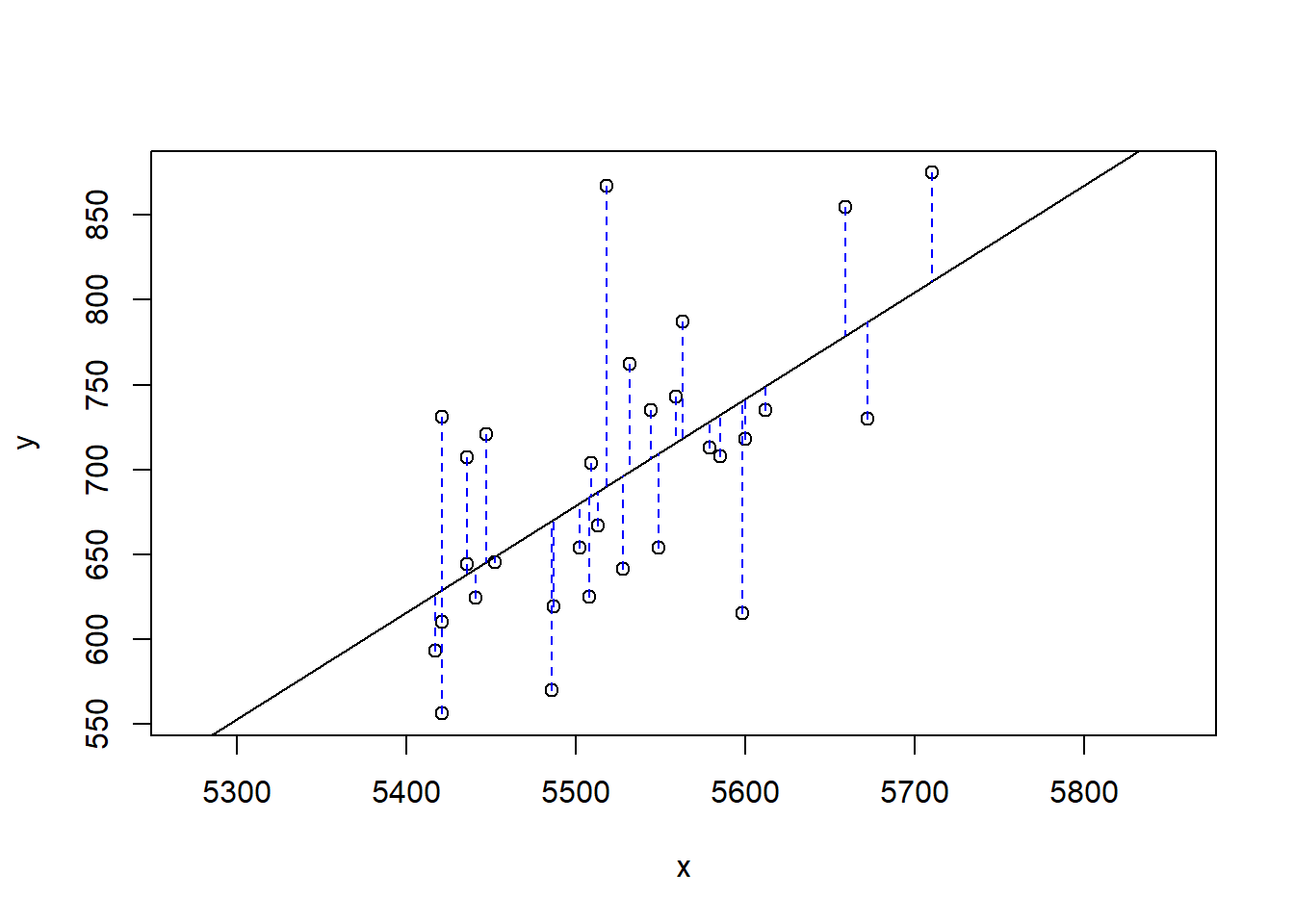## Click two points to make a line.

## Call:
## lm(formula = y ~ x, data = pts)
##
## Coefficients:
## (Intercept)            x
##  -2789.2429       0.6305
##
## Sum of Squares:  123721.9

After running this command, you’ll be prompted to click two points on the plot to define a line. Once you’ve done that, the line you specified will be shown in black and the residuals in blue. Note that there are 30 residuals, one for each of the 30 observations. Recall that the residuals are the difference between the observed values and the values predicted by the line:

$e_i = y_i - \hat{y}_i$

The most common way to do linear regression is to select the line that minimizes the sum of squared residuals. To visualize the squared residuals, you can rerun the plot command and add the argument showSquares = TRUE.

plot_ss(x = mlb11$at_bats, y = mlb11$runs, showSquares = TRUE)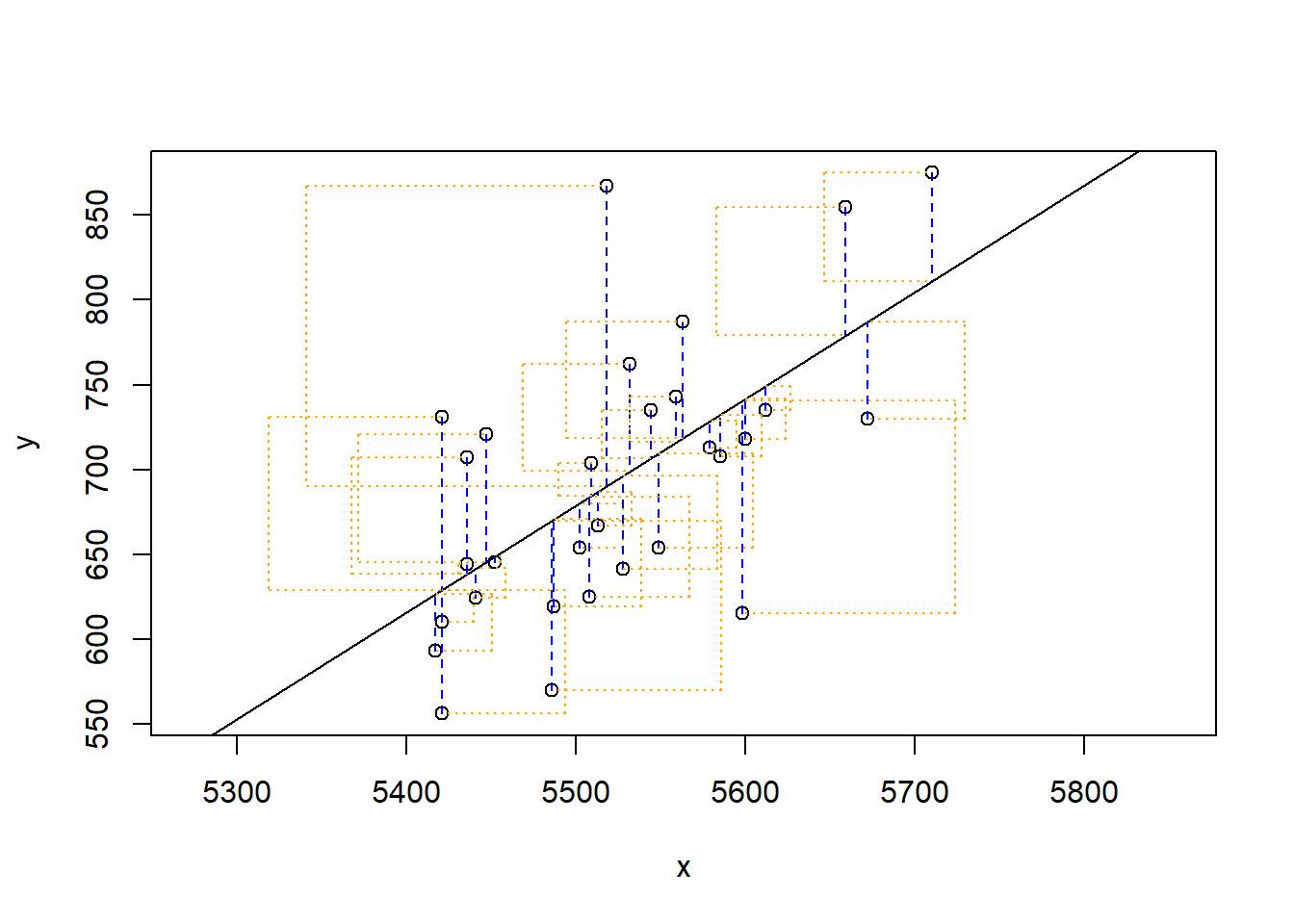## Click two points to make a line.

## Call:
## lm(formula = y ~ x, data = pts)
##
## Coefficients:
## (Intercept)            x
##  -2789.2429       0.6305
##
## Sum of Squares:  123721.9

Note that the output from the plot_ss function provides you with the slope and intercept of your line as well as the sum of squares.

1. Using plot_ss, choose a line that does a good job of minimizing the sum of squares. Run the function several times. What was the smallest sum of squares that you got? How does it compare to your neighbors?

The smallest sum of squares found was 123721.9

## The linear model

It is rather cumbersome to try to get the correct least squares line, i.e. the line that minimizes the sum of squared residuals, through trial and error. Instead we can use the lm function in R to fit the linear model (a.k.a. regression line).

m1 <- lm(runs ~ at_bats, data = mlb11)

The first argument in the function lm is a formula that takes the form y ~ x. Here it can be read that we want to make a linear model of runs as a function of at_bats. The second argument specifies that R should look in the mlb11 data frame to find the runs and at_bats variables.

The output of lm is an object that contains all of the information we need about the linear model that was just fit. We can access this information using the summary function.

summary(m1)
##
## Call:
## lm(formula = runs ~ at_bats, data = mlb11)
##
## Residuals:
##     Min      1Q  Median      3Q     Max
## -125.58  -47.05  -16.59   54.40  176.87
##
## Coefficients:
##               Estimate Std. Error t value Pr(>|t|)
## (Intercept) -2789.2429   853.6957  -3.267 0.002871 **
## at_bats         0.6305     0.1545   4.080 0.000339 ***
## ---
## Signif. codes:  0 '***' 0.001 '**' 0.01 '*' 0.05 '.' 0.1 ' ' 1
##
## Residual standard error: 66.47 on 28 degrees of freedom
## Multiple R-squared:  0.3729, Adjusted R-squared:  0.3505
## F-statistic: 16.65 on 1 and 28 DF,  p-value: 0.0003388

Let’s consider this output piece by piece. First, the formula used to describe the model is shown at the top. After the formula you find the five-number summary of the residuals. The “Coefficients” table shown next is key; its first column displays the linear model’s y-intercept and the coefficient of at_bats. With this table, we can write down the least squares regression line for the linear model:

$\hat{y} = -2789.2429 + 0.6305 * atbats$

One last piece of information we will discuss from the summary output is the Multiple R-squared, or more simply, $$R^2$$. The $$R^2$$ value represents the proportion of variability in the response variable that is explained by the explanatory variable. For this model, 37.3% of the variability in runs is explained by at-bats.

1. Fit a new model that uses homeruns to predict runs. Using the estimates from the R output, write the equation of the regression line. What does the slope tell us in the context of the relationship between success of a team and its home runs?
plot_ss(x = mlb11$homeruns, y = mlb11$runs, showSquares = TRUE)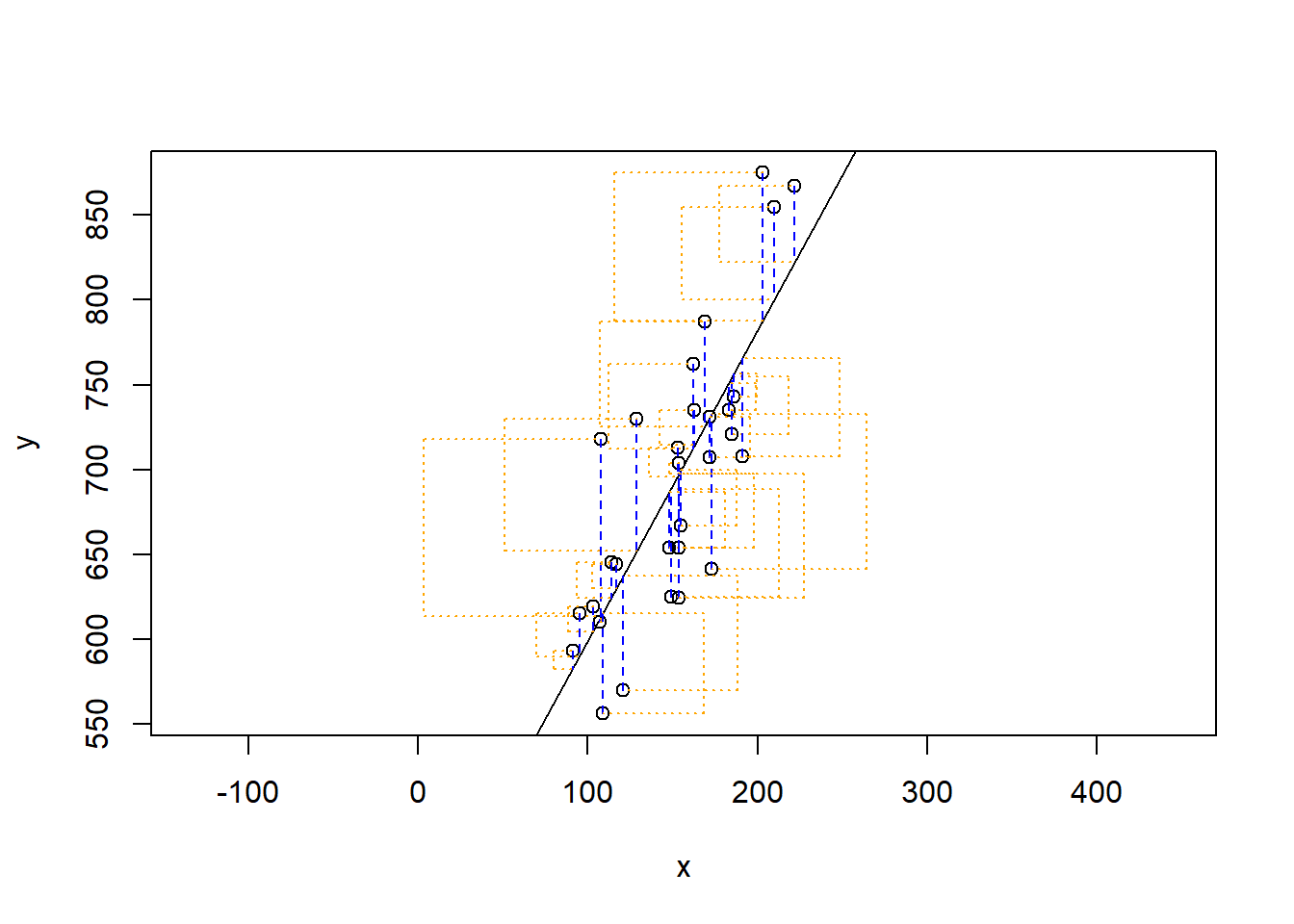## Click two points to make a line.

## Call:
## lm(formula = y ~ x, data = pts)
##
## Coefficients:
## (Intercept)            x
##     415.239        1.835
##
## Sum of Squares:  73671.99
cor(mlb11$runs, mlb11$homeruns)
##  0.7915577
m2 <- lm(runs ~ homeruns, data = mlb11)

summary(m2)
##
## Call:
## lm(formula = runs ~ homeruns, data = mlb11)
##
## Residuals:
##     Min      1Q  Median      3Q     Max
## -91.615 -33.410   3.231  24.292 104.631
##
## Coefficients:
##             Estimate Std. Error t value Pr(>|t|)
## (Intercept) 415.2389    41.6779   9.963 1.04e-10 ***
## homeruns      1.8345     0.2677   6.854 1.90e-07 ***
## ---
## Signif. codes:  0 '***' 0.001 '**' 0.01 '*' 0.05 '.' 0.1 ' ' 1
##
## Residual standard error: 51.29 on 28 degrees of freedom
## Multiple R-squared:  0.6266, Adjusted R-squared:  0.6132
## F-statistic: 46.98 on 1 and 28 DF,  p-value: 1.9e-07

Regrssion line equation is: runs = 1.8345.homeruns + 415.2389

In this case, the slope tells us that the more homeruns, the more runs the team will have, therfore increasing the chances of the team winning games.

## Prediction and prediction errors

Let’s create a scatterplot with the least squares line laid on top.

plot(mlb11$runs ~ mlb11$at_bats)
abline(m1)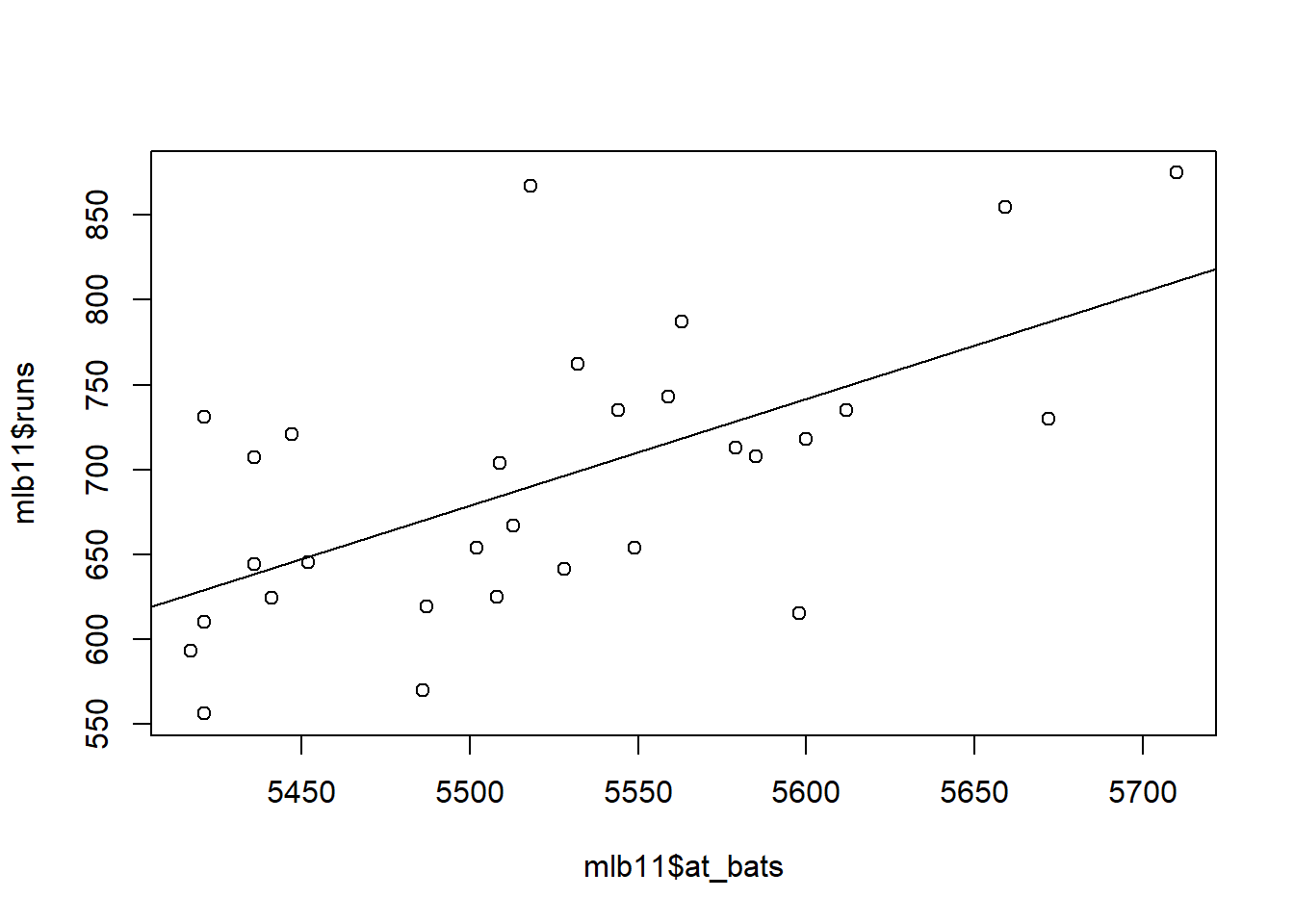The function abline plots a line based on its slope and intercept. Here, we used a shortcut by providing the model m1, which contains both parameter estimates. This line can be used to predict $$y$$ at any value of $$x$$. When predictions are made for values of $$x$$ that are beyond the range of the observed data, it is referred to as extrapolation and is not usually recommended. However, predictions made within the range of the data are more reliable. They’re also used to compute the residuals.

1. If a team manager saw the least squares regression line and not the actual data, how many runs would he or she predict for a team with 5,578 at-bats? Is this an overestimate or an underestimate, and by how much? In other words, what is the residual for this prediction?

Since the regression line is y = 0.6305⋅x − 2789.2429

runs <- function(x = NULL)
{
y <- 0.6305 * x - 2789.2429
y <- round(y,0)
return(y)
}

y <- runs(5578)
y
##  728
y - subset(mlb11, at_bats==5578+1, select = runs)
##    runs
## 16   15

Since the manager didn’t look at the data by doing these calculations, he can get a totals of 728.

Since the real nearest point from the table is totaled at 713 from Philadelphia Phillies with at bats of 5579, the difference will be of 15, resulting in an over estimation of 15 runs.

## Model diagnostics

To assess whether the linear model is reliable, we need to check for (1) linearity, (2) nearly normal residuals, and (3) constant variability.

Linearity: You already checked if the relationship between runs and at-bats is linear using a scatterplot. We should also verify this condition with a plot of the residuals vs. at-bats. Recall that any code following a # is intended to be a comment that helps understand the code but is ignored by R.

plot(m1$residuals ~ mlb11$at_bats)
abline(h = 0, lty = 3)  # adds a horizontal dashed line at y = 0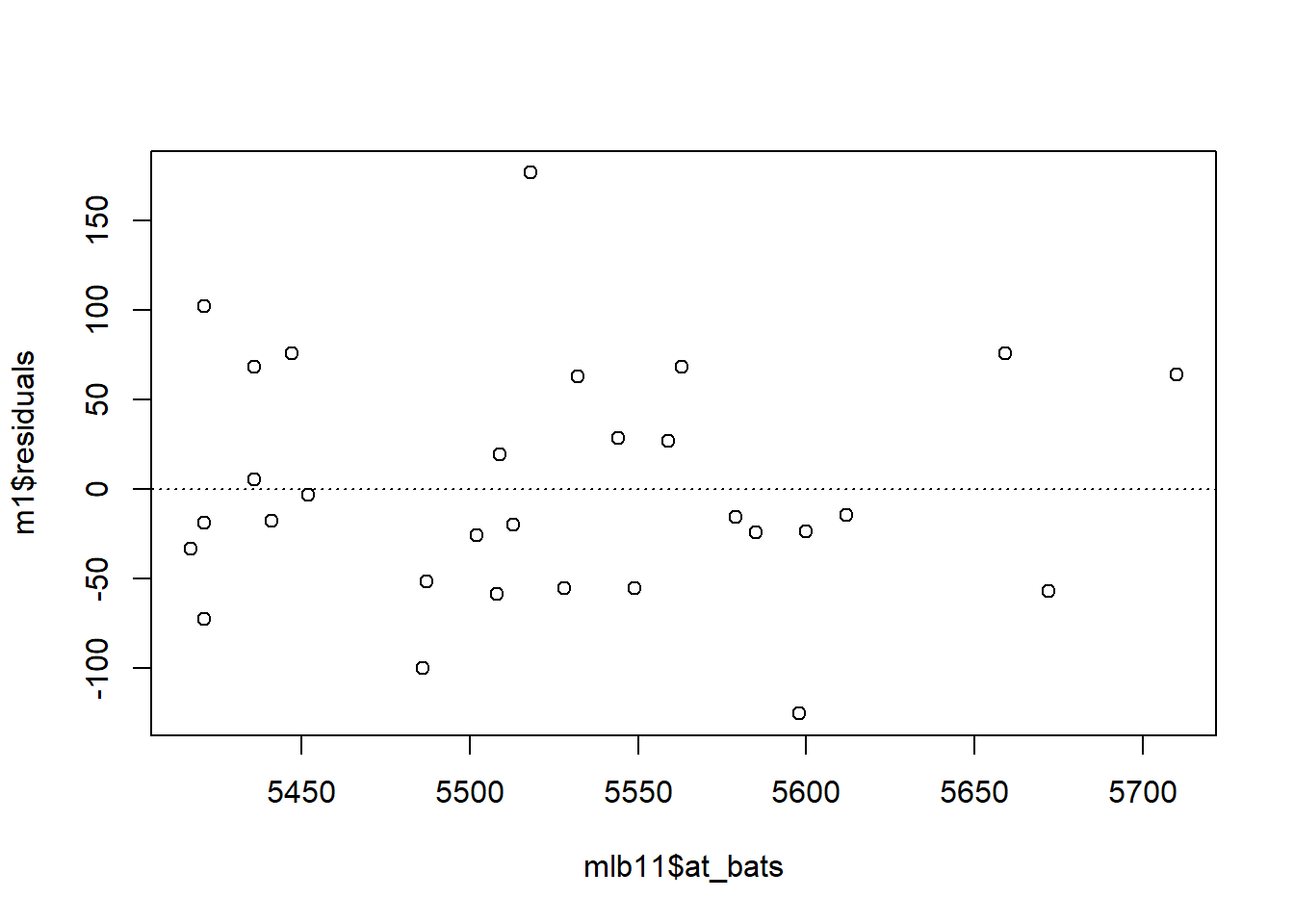1. Is there any apparent pattern in the residuals plot? What does this indicate about the linearity of the relationship between runs and at-bats?

We do notice that the residuals are positioned around zero, indicating a constant linearity of the relationship between runs and at_bats.

Nearly normal residuals: To check this condition, we can look at a histogram

hist(m1$residuals)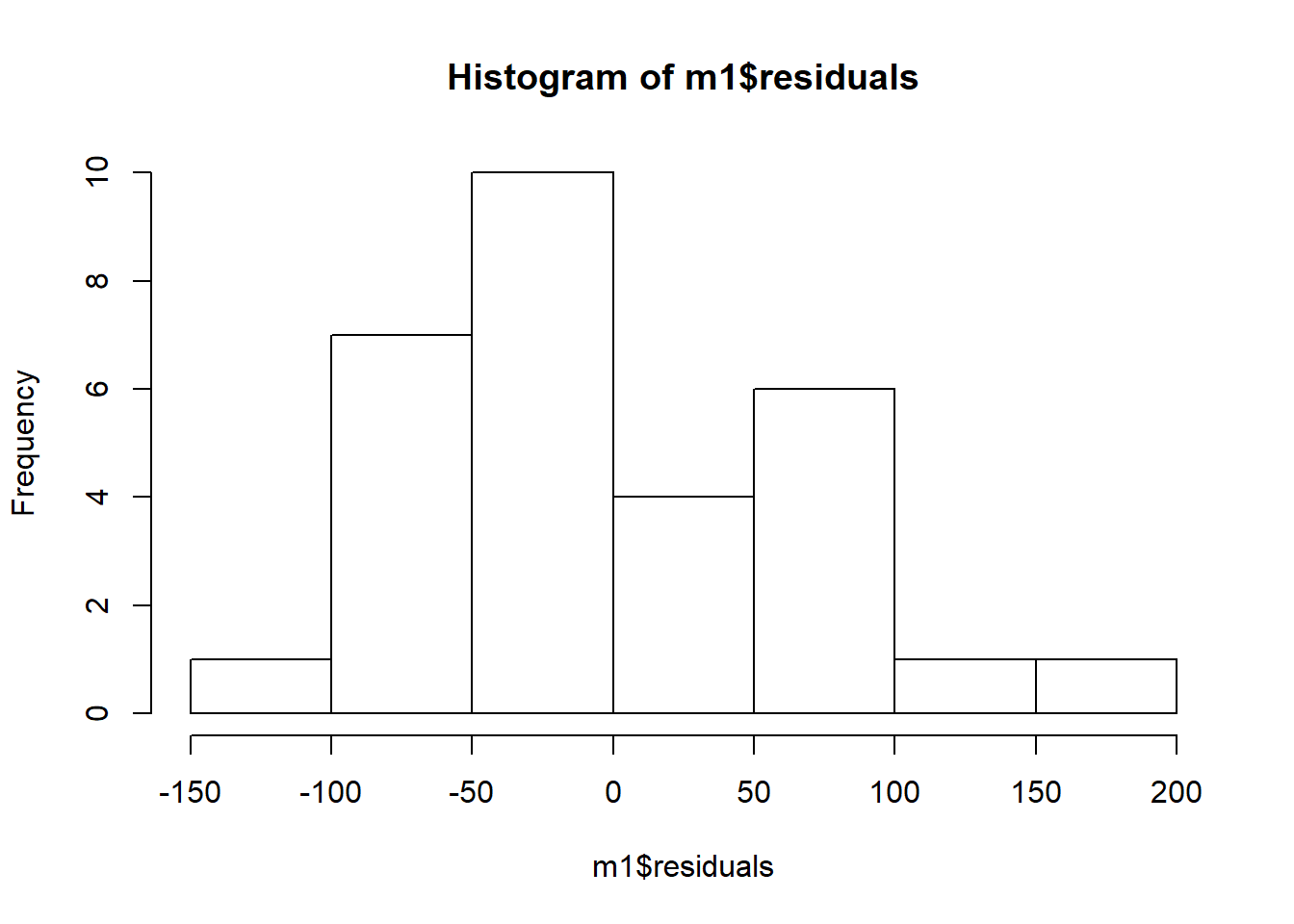or a normal probability plot of the residuals. qqnorm(m1$residuals)
qqline(m1$residuals) # adds diagonal line to the normal prob plot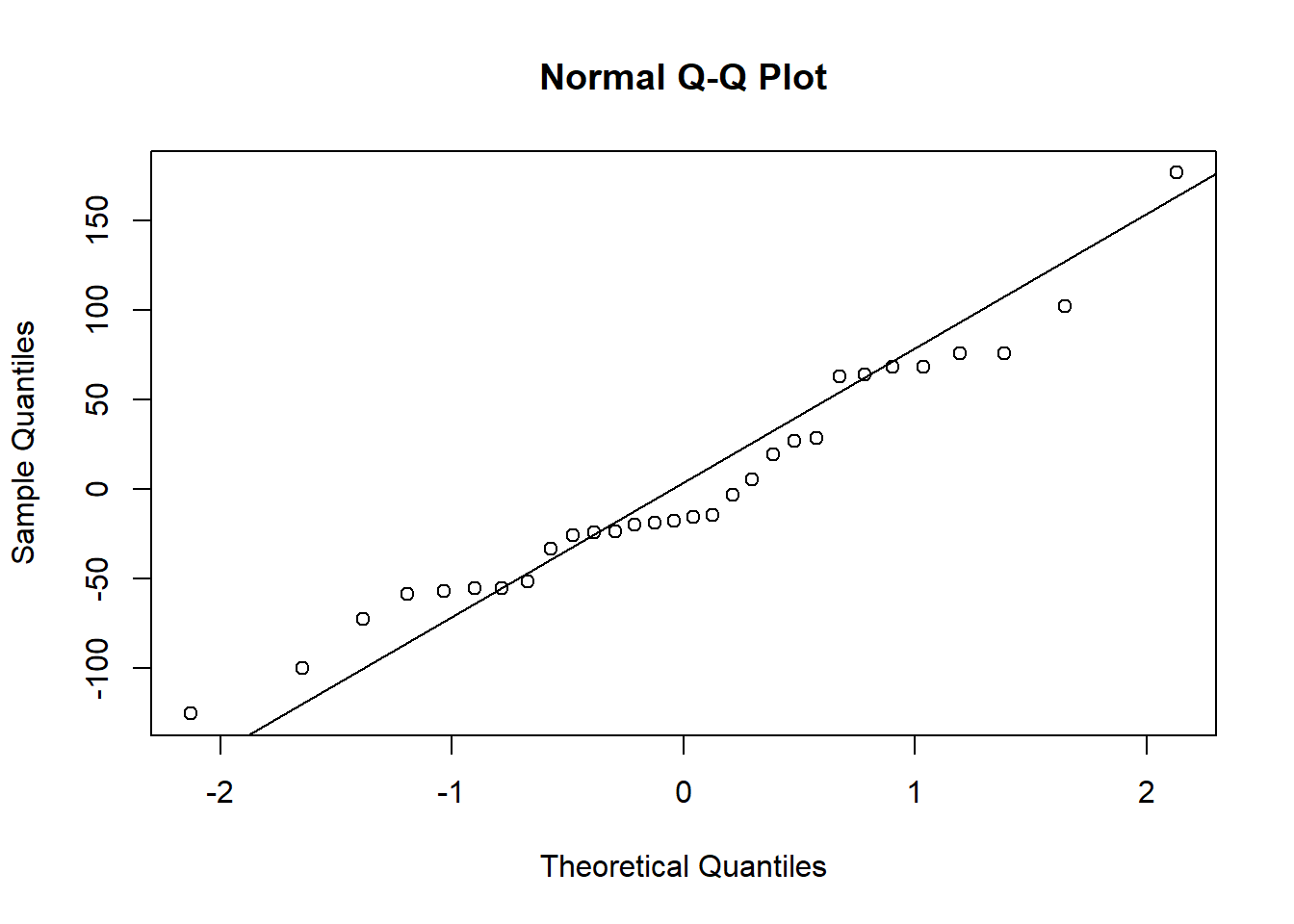1. Based on the histogram and the normal probability plot, does the nearly normal residuals condition appear to be met? Looking at both graphs, we can see that the normal residuals condition seems to be met. Constant variability: 1. Based on the plot in (1), does the constant variability condition appear to be met? Based on the plot in (1), the points seem to follow a pattern, making constant variability condition to be met. ## On Your Own • Choose another traditional variable from mlb11 that you think might be a good predictor of runs. Produce a scatterplot of the two variables and fit a linear model. At a glance, does there seem to be a linear relationship? Choosing Hits variable: m3 <- lm(runs ~ hits, data = mlb11) plot(mlb11$hits, mlb11$runs, # plot the variables xlab="Hits", # x−axis label ylab="Runs") # y−axis label abline(m3)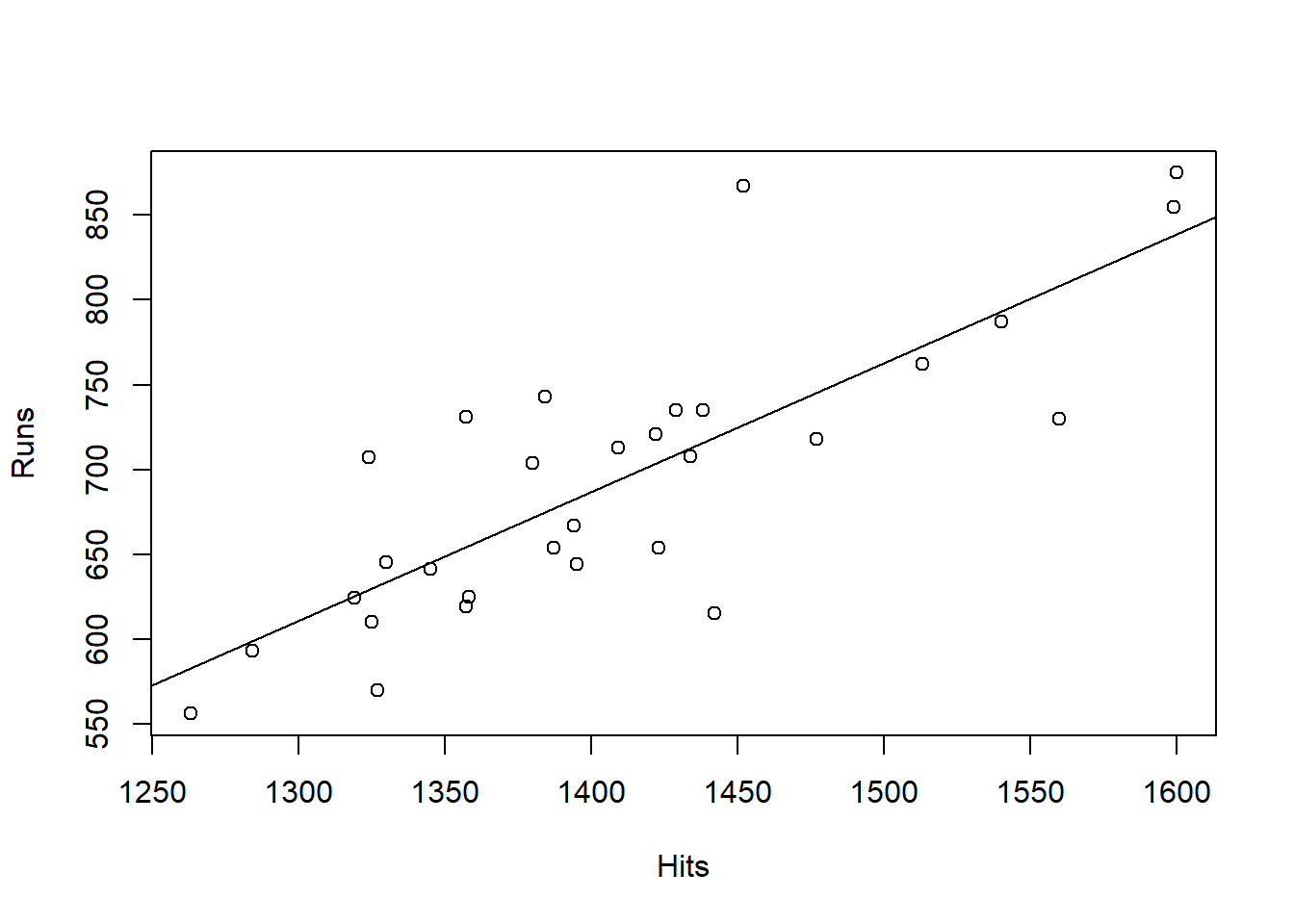cor(mlb11$runs, mlb11$hits) ##  0.8012108 At a glance, there seems to be a linear positive relationship. • How does this relationship compare to the relationship between runs and at_bats? Use the R$$^2$$ values from the two model summaries to compare. Does your variable seem to predict runs better than at_bats? How can you tell? plot_ss(x = mlb11$hits, y = mlb11$runs, showSquares = TRUE)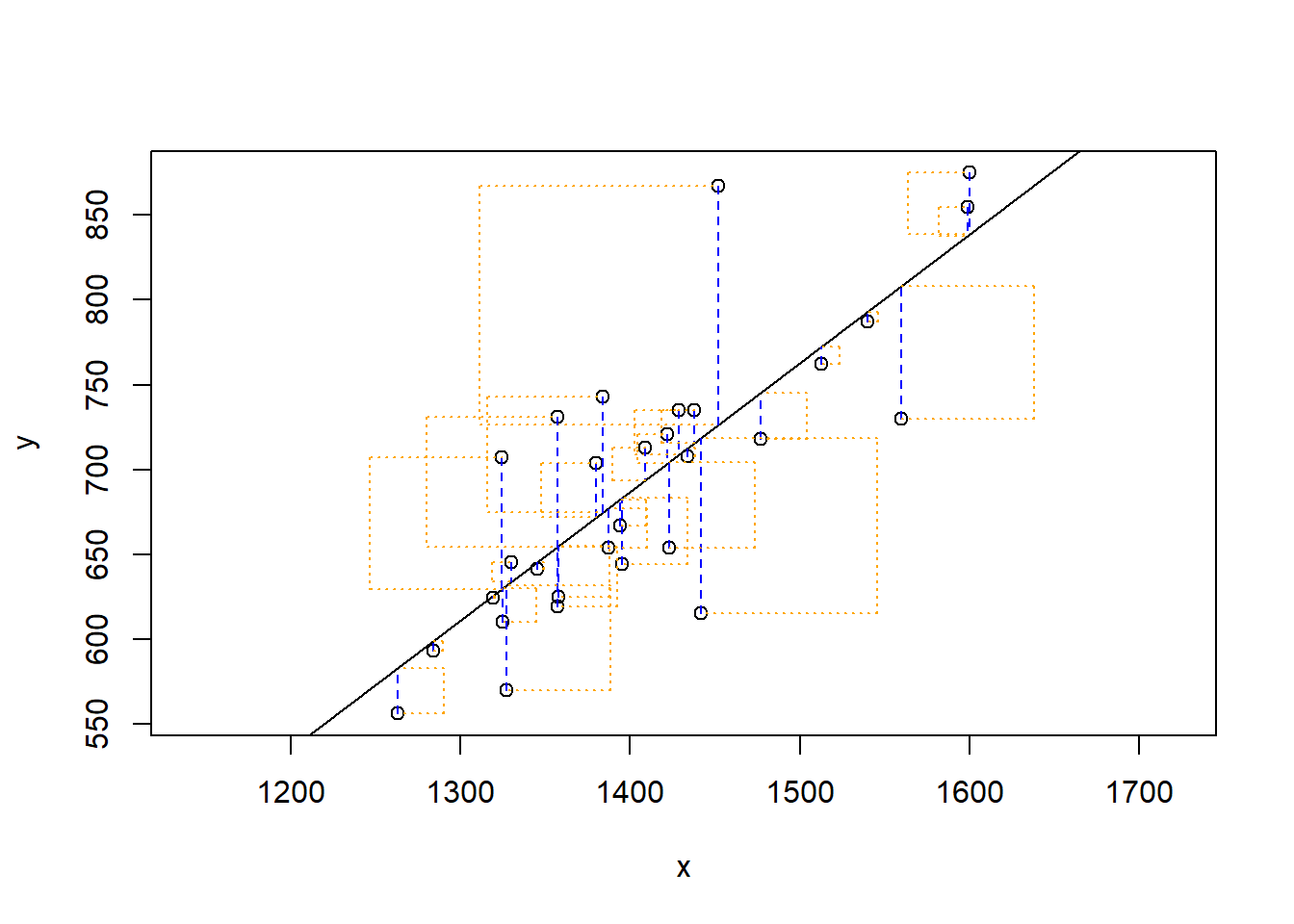## Click two points to make a line. ## Call: ## lm(formula = y ~ x, data = pts) ## ## Coefficients: ## (Intercept) x ## -375.5600 0.7589 ## ## Sum of Squares: 70638.75 m4 <- lm(runs ~ hits, data = mlb11) summary(m4) ## ## Call: ## lm(formula = runs ~ hits, data = mlb11) ## ## Residuals: ## Min 1Q Median 3Q Max ## -103.718 -27.179 -5.233 19.322 140.693 ## ## Coefficients: ## Estimate Std. Error t value Pr(>|t|) ## (Intercept) -375.5600 151.1806 -2.484 0.0192 * ## hits 0.7589 0.1071 7.085 1.04e-07 *** ## --- ## Signif. codes: 0 '***' 0.001 '**' 0.01 '*' 0.05 '.' 0.1 ' ' 1 ## ## Residual standard error: 50.23 on 28 degrees of freedom ## Multiple R-squared: 0.6419, Adjusted R-squared: 0.6292 ## F-statistic: 50.2 on 1 and 28 DF, p-value: 1.043e-07 For this model, 64.19% of the variability in runs is explained by Hits. Looking at the results from the at_bats in which only 34.3% was explained by it, we can conclude that this model seem to predict runs better. • Now that you can summarize the linear relationship between two variables, investigate the relationships between runs and each of the other five traditional variables. Which variable best predicts runs? Support your conclusion using the graphical and numerical methods we’ve discussed (for the sake of conciseness, only include output for the best variable, not all five). ### A- runs & homeruns plot_ss(x = mlb11$homeruns, y = mlb11$runs, showSquares = TRUE)## Click two points to make a line. ## Call: ## lm(formula = y ~ x, data = pts) ## ## Coefficients: ## (Intercept) x ## 415.239 1.835 ## ## Sum of Squares: 73671.99 cor(mlb11$runs, mlb11$homeruns) ##  0.7915577 Correlation: 0.7915577 rm1 <- lm(runs ~ homeruns, data = mlb11) summary(rm1) ## ## Call: ## lm(formula = runs ~ homeruns, data = mlb11) ## ## Residuals: ## Min 1Q Median 3Q Max ## -91.615 -33.410 3.231 24.292 104.631 ## ## Coefficients: ## Estimate Std. Error t value Pr(>|t|) ## (Intercept) 415.2389 41.6779 9.963 1.04e-10 *** ## homeruns 1.8345 0.2677 6.854 1.90e-07 *** ## --- ## Signif. codes: 0 '***' 0.001 '**' 0.01 '*' 0.05 '.' 0.1 ' ' 1 ## ## Residual standard error: 51.29 on 28 degrees of freedom ## Multiple R-squared: 0.6266, Adjusted R-squared: 0.6132 ## F-statistic: 46.98 on 1 and 28 DF, p-value: 1.9e-07 r2_1 <- c("0.6266") ### B- runs & bat_avg plot_ss(x = mlb11$bat_avg, y = mlb11$runs, showSquares = TRUE)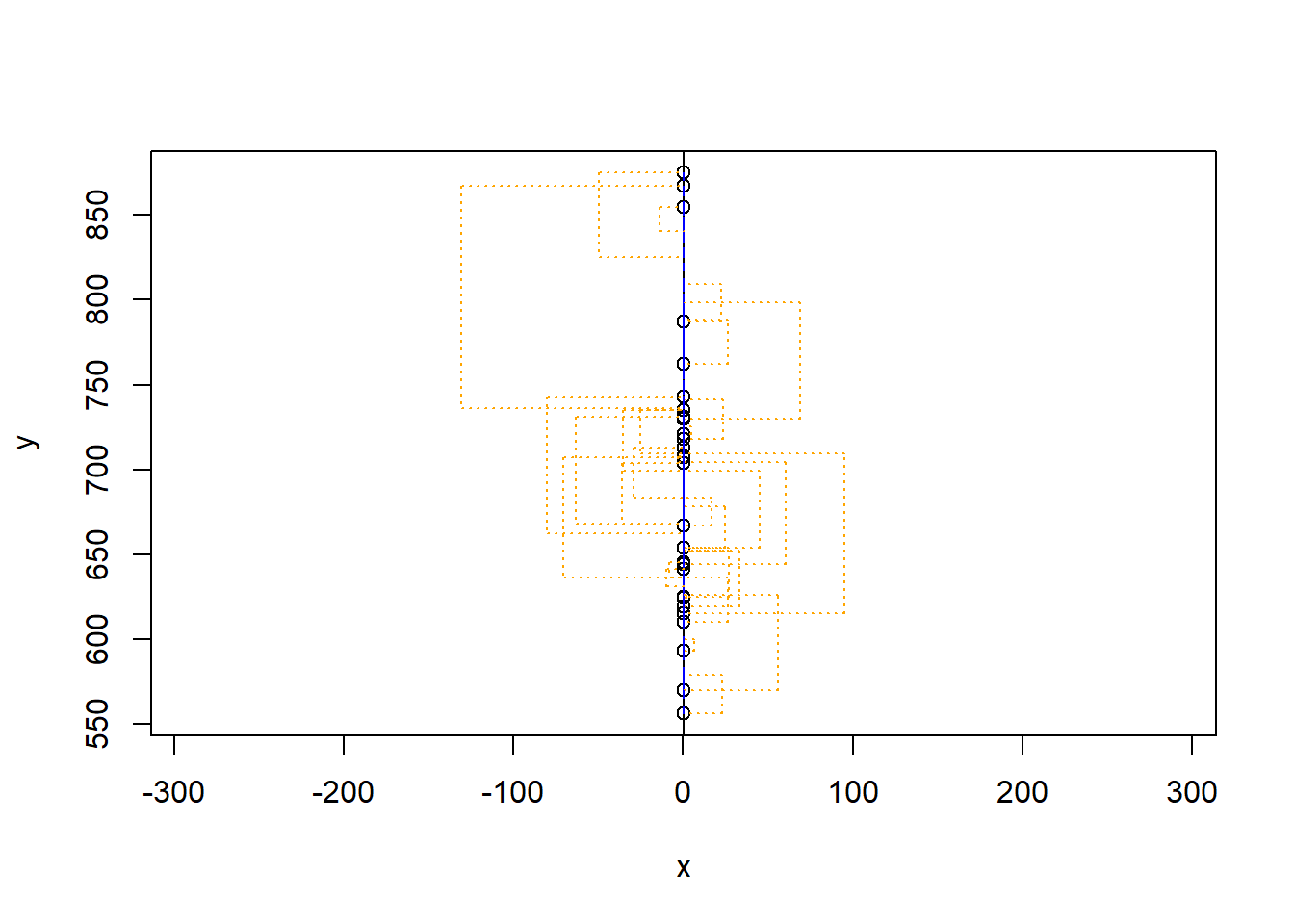## Click two points to make a line. ## Call: ## lm(formula = y ~ x, data = pts) ## ## Coefficients: ## (Intercept) x ## -642.8 5242.2 ## ## Sum of Squares: 67849.52 cor(mlb11$runs, mlb11$bat_avg) ##  0.8099859 Correlation is: 0.8099859 rm2 <- lm(runs ~ bat_avg, data = mlb11) summary(rm2) ## ## Call: ## lm(formula = runs ~ bat_avg, data = mlb11) ## ## Residuals: ## Min 1Q Median 3Q Max ## -94.676 -26.303 -5.496 28.482 131.113 ## ## Coefficients: ## Estimate Std. Error t value Pr(>|t|) ## (Intercept) -642.8 183.1 -3.511 0.00153 ** ## bat_avg 5242.2 717.3 7.308 5.88e-08 *** ## --- ## Signif. codes: 0 '***' 0.001 '**' 0.01 '*' 0.05 '.' 0.1 ' ' 1 ## ## Residual standard error: 49.23 on 28 degrees of freedom ## Multiple R-squared: 0.6561, Adjusted R-squared: 0.6438 ## F-statistic: 53.41 on 1 and 28 DF, p-value: 5.877e-08 r2_2 <- c("0.6561") hist(rm2$residuals)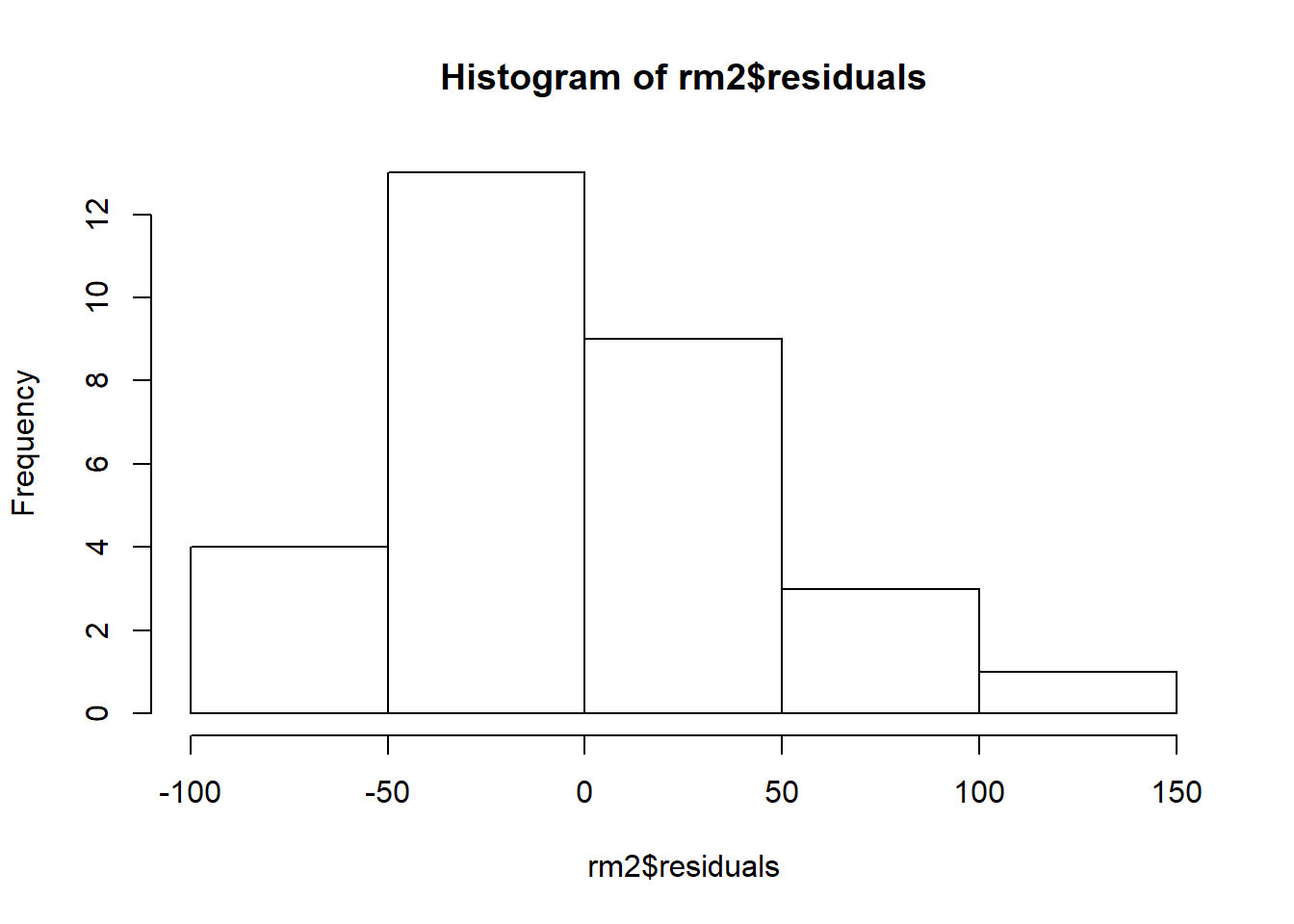qqnorm(rm2$residuals) qqline(rm2$residuals)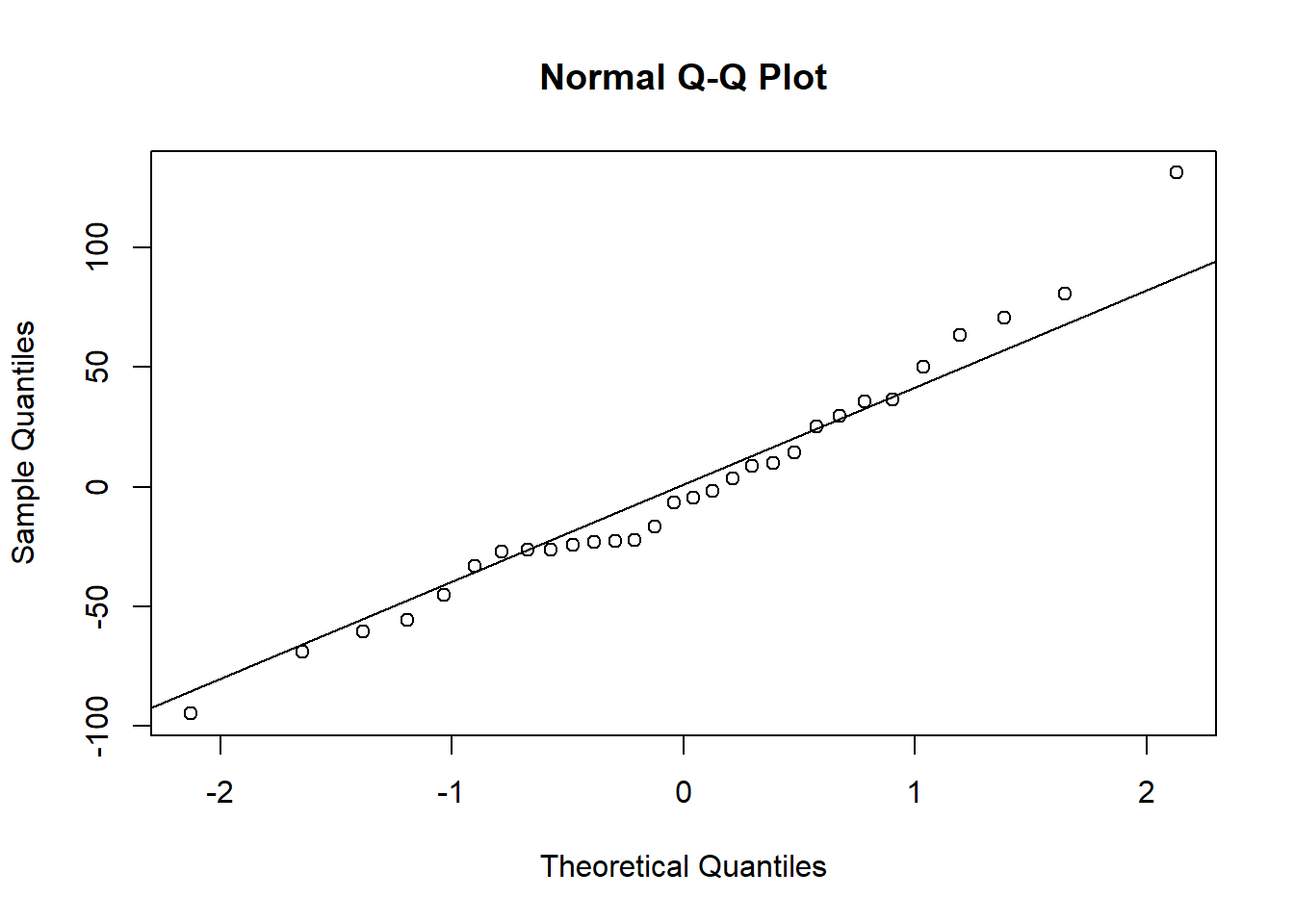### C- runs & strikeouts

plot_ss(x = mlb11$strikeouts, y = mlb11$runs, showSquares = TRUE)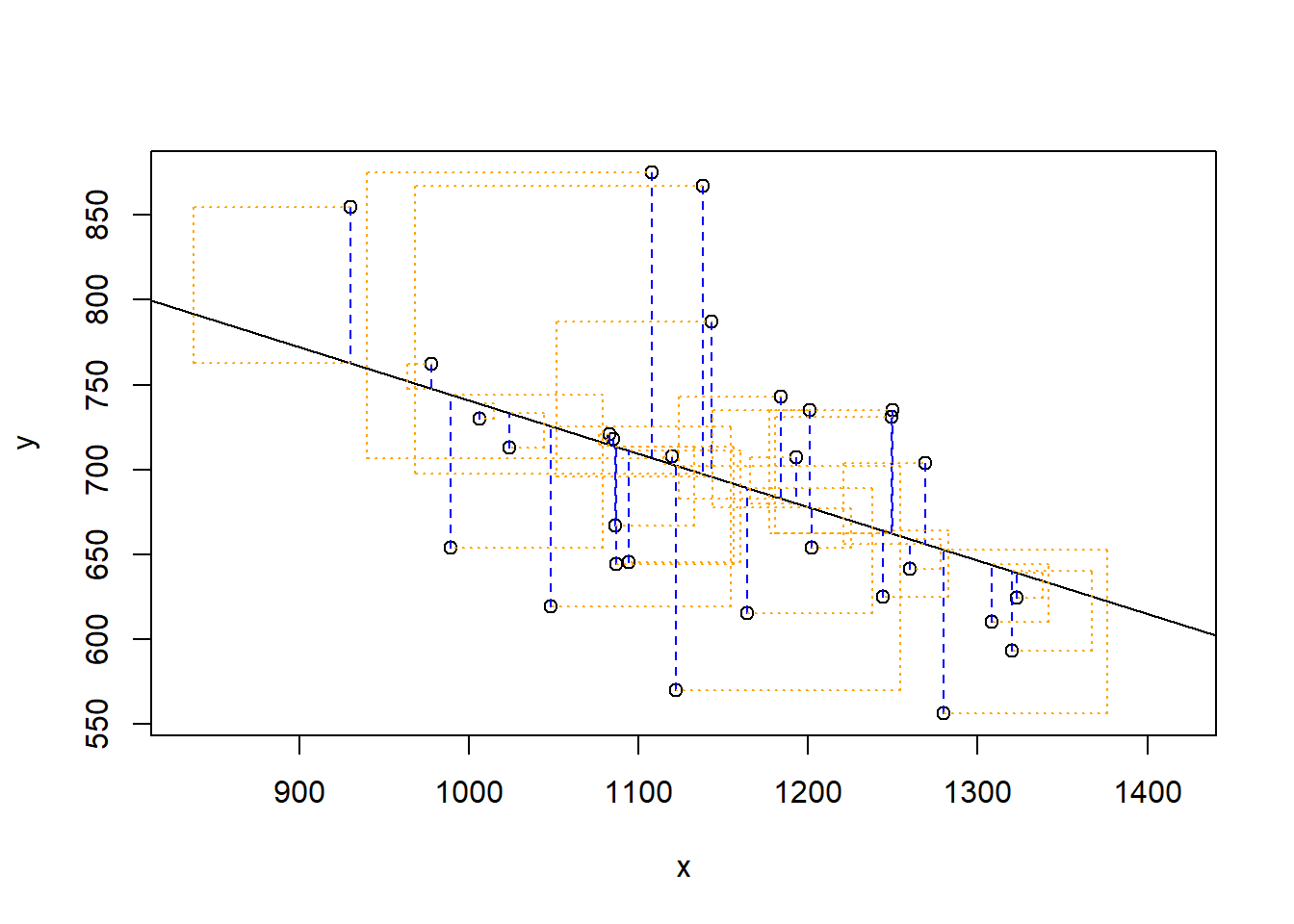## Click two points to make a line.

## Call:
## lm(formula = y ~ x, data = pts)
##
## Coefficients:
## (Intercept)            x
##   1054.7342      -0.3141
##
## Sum of Squares:  163870.1
cor(mlb11$runs, mlb11$strikeouts)
##  -0.4115312

Correlation is: -0.4115312

rm3 <- lm(runs ~ strikeouts, data = mlb11)
summary(rm3)
##
## Call:
## lm(formula = runs ~ strikeouts, data = mlb11)
##
## Residuals:
##     Min      1Q  Median      3Q     Max
## -132.27  -46.95  -11.92   55.14  169.76
##
## Coefficients:
##              Estimate Std. Error t value Pr(>|t|)
## (Intercept) 1054.7342   151.7890   6.949 1.49e-07 ***
## strikeouts    -0.3141     0.1315  -2.389   0.0239 *
## ---
## Signif. codes:  0 '***' 0.001 '**' 0.01 '*' 0.05 '.' 0.1 ' ' 1
##
## Residual standard error: 76.5 on 28 degrees of freedom
## Multiple R-squared:  0.1694, Adjusted R-squared:  0.1397
## F-statistic: 5.709 on 1 and 28 DF,  p-value: 0.02386
r2_3 <- c("0.1694")

### D- runs & stolen_bases

plot_ss(x = mlb11$stolen_bases, y = mlb11$runs, showSquares = TRUE)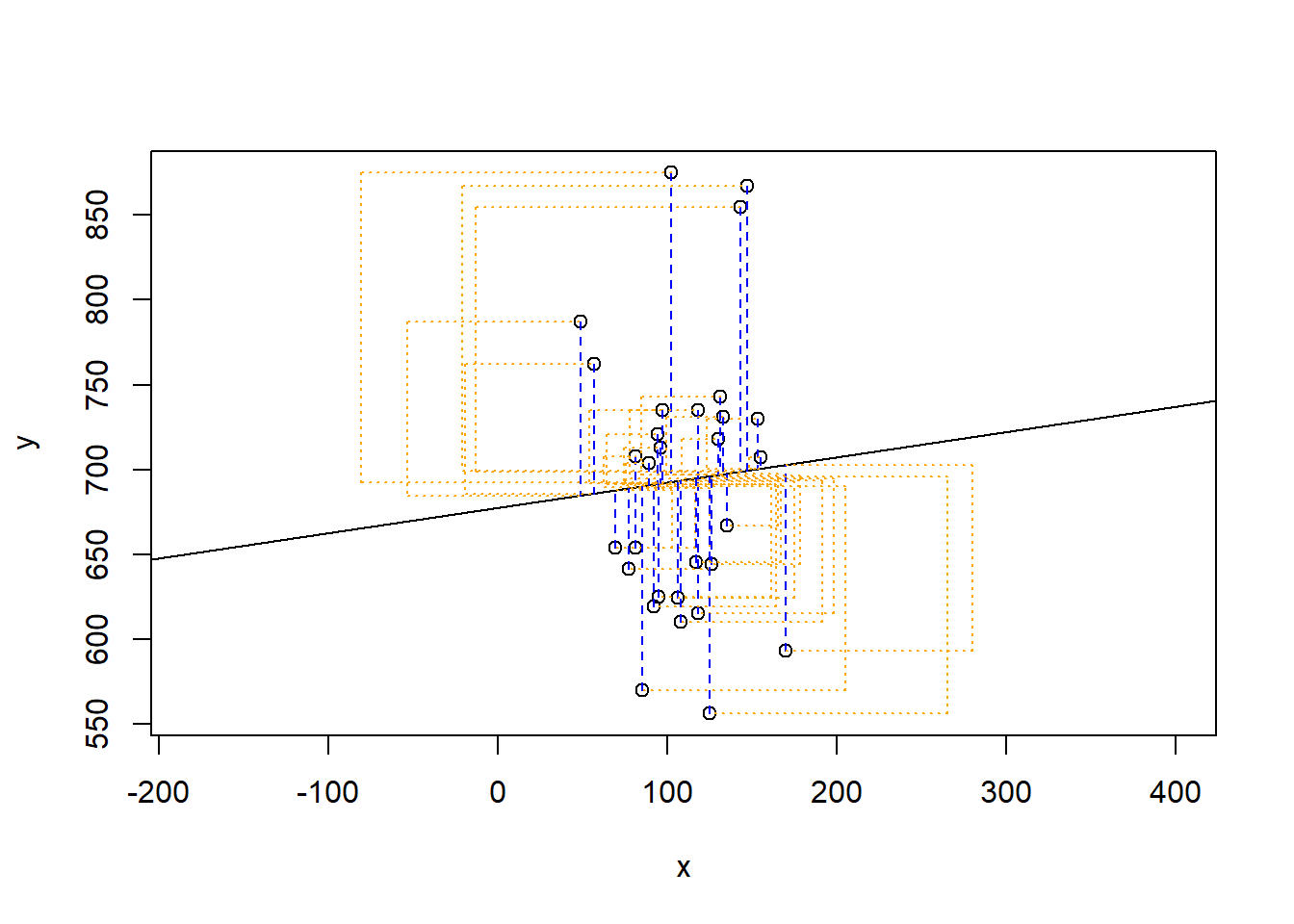## Click two points to make a line.

## Call:
## lm(formula = y ~ x, data = pts)
##
## Coefficients:
## (Intercept)            x
##    677.3074       0.1491
##
## Sum of Squares:  196706.3
cor(mlb11$runs, mlb11$stolen_bases)
##  0.05398141

Correlation: 0.05398141

rm4 <- lm(runs ~ stolen_bases, data = mlb11)
summary(rm4)
##
## Call:
## lm(formula = runs ~ stolen_bases, data = mlb11)
##
## Residuals:
##     Min      1Q  Median      3Q     Max
## -139.94  -62.87   10.01   38.54  182.49
##
## Coefficients:
##              Estimate Std. Error t value Pr(>|t|)
## (Intercept)  677.3074    58.9751  11.485 4.17e-12 ***
## stolen_bases   0.1491     0.5211   0.286    0.777
## ---
## Signif. codes:  0 '***' 0.001 '**' 0.01 '*' 0.05 '.' 0.1 ' ' 1
##
## Residual standard error: 83.82 on 28 degrees of freedom
## Multiple R-squared:  0.002914,   Adjusted R-squared:  -0.0327
## F-statistic: 0.08183 on 1 and 28 DF,  p-value: 0.7769
r2_4 <- c("0.002914")

### E- runs & wins

plot_ss(x = mlb11$wins, y = mlb11$runs, showSquares = TRUE)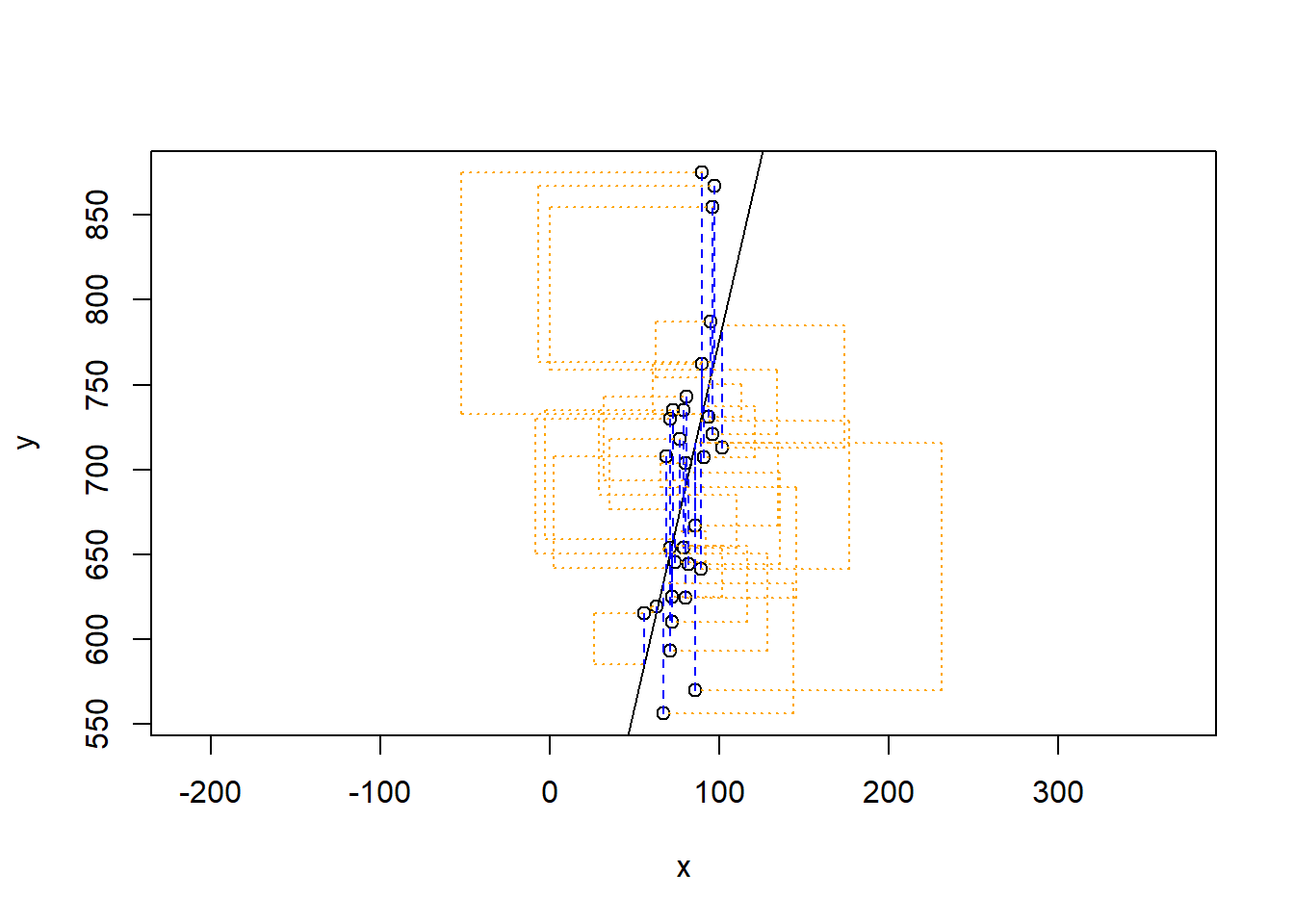## Click two points to make a line.

## Call:
## lm(formula = y ~ x, data = pts)
##
## Coefficients:
## (Intercept)            x
##     342.121        4.341
##
## Sum of Squares:  126068.4
cor(mlb11$runs, mlb11$wins)
##  0.6008088

Correlation: 0.6008088

rm5 <- lm(runs ~ wins, data = mlb11)
summary(rm5)
##
## Call:
## lm(formula = runs ~ wins, data = mlb11)
##
## Residuals:
##      Min       1Q   Median       3Q      Max
## -145.450  -47.506   -7.482   47.346  142.186
##
## Coefficients:
##             Estimate Std. Error t value Pr(>|t|)
## (Intercept)  342.121     89.223   3.834 0.000654 ***
## wins           4.341      1.092   3.977 0.000447 ***
## ---
## Signif. codes:  0 '***' 0.001 '**' 0.01 '*' 0.05 '.' 0.1 ' ' 1
##
## Residual standard error: 67.1 on 28 degrees of freedom
## Multiple R-squared:  0.361,  Adjusted R-squared:  0.3381
## F-statistic: 15.82 on 1 and 28 DF,  p-value: 0.0004469
r2_5 <- c("0.361")

### Best Approach:

r2 <- c(r2_1, r2_2, r2_3, r2_4, r2_5)
r2 <- data.frame(r2, stringsAsFactors = TRUE)

r2
##         r2
## 1   0.6266
## 2   0.6561
## 3   0.1694
## 4 0.002914
## 5    0.361

From the table above, we can see that the best approach is by selecting bat_avg.

• Now examine the three newer variables. These are the statistics used by the author of Moneyball to predict a teams success. In general, are they more or less effective at predicting runs that the old variables? Explain using appropriate graphical and numerical evidence. Of all ten variables we’ve analyzed, which seems to be the best predictor of runs? Using the limited (or not so limited) information you know about these baseball statistics, does your result make sense?

### A- runs & new_onbase

plot_ss(x = mlb11$new_onbase, y = mlb11$runs, showSquares = TRUE)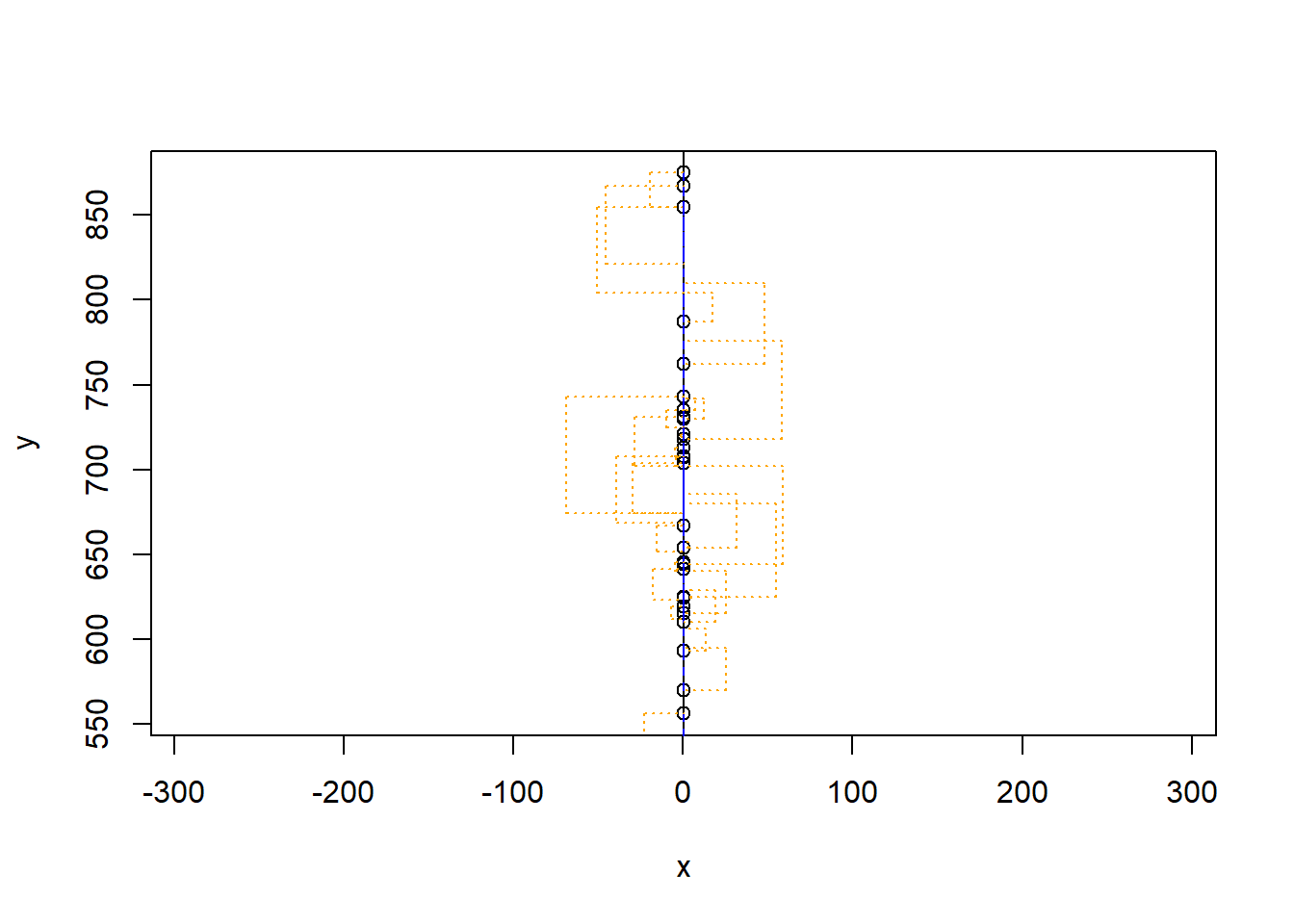## Click two points to make a line.

## Call:
## lm(formula = y ~ x, data = pts)
##
## Coefficients:
## (Intercept)            x
##       -1118         5654
##
## Sum of Squares:  29768.7
cor1 <- cor(mlb11$runs, mlb11$new_onbase)
cor1
##  0.9214691
rm1 <- lm(runs ~ new_onbase, data = mlb11)
summary(rm1)
##
## Call:
## lm(formula = runs ~ new_onbase, data = mlb11)
##
## Residuals:
##     Min      1Q  Median      3Q     Max
## -58.270 -18.335   3.249  19.520  69.002
##
## Coefficients:
##             Estimate Std. Error t value Pr(>|t|)
## (Intercept)  -1118.4      144.5  -7.741 1.97e-08 ***
## new_onbase    5654.3      450.5  12.552 5.12e-13 ***
## ---
## Signif. codes:  0 '***' 0.001 '**' 0.01 '*' 0.05 '.' 0.1 ' ' 1
##
## Residual standard error: 32.61 on 28 degrees of freedom
## Multiple R-squared:  0.8491, Adjusted R-squared:  0.8437
## F-statistic: 157.6 on 1 and 28 DF,  p-value: 5.116e-13
r2_6 <- c("0.8491")

hist(rm1$residuals)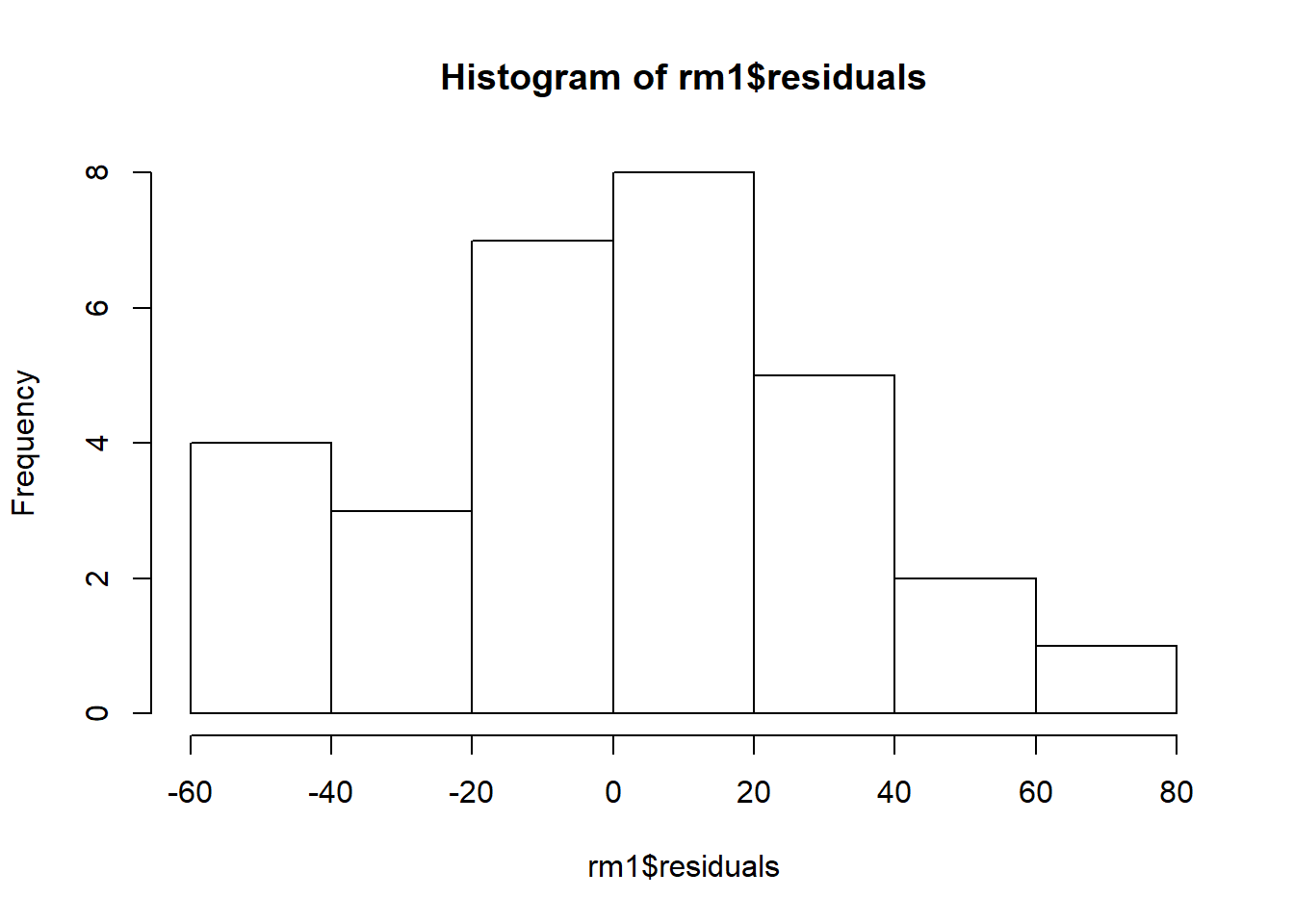qqnorm(rm1$residuals)